HTML5 表单元素

HTML5 有以下新的表单元素:

HTML5 <datalist> 元素

<datalist> 元素规定输入域的选项列表。

<datalist> 属性规定 form 或 input 域应该拥有自动完成功能。当用户在自动完成域中开始输入时，浏览器应该在该域中显示填写的选项：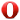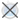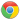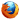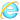实例

<input> 元素使用<datalist>预定义值:

<input list="browsers">

<datalist id="browsers">
<option value="Internet Explorer">
<option value="Firefox">
<option value="Chrome">
<option value="Opera">
<option value="Safari">
</datalist>

HTML5 <keygen> 元素

<keygen> 元素的作用是提供一种验证用户的可靠方法。

<keygen>标签规定用于表单的密钥对生成器字段。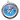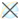实例

<form action="demo_keygen.asp" method="get">

<input type="submit">
</form>

HTML5 <output> 元素

<output> 元素用于不同类型的输出，比如计算或脚本输出：实例

<form oninput="x.value=parseInt(a.value)+parseInt(b.value)">0
<input type="range" id="a" value="50">100 +
<input type="number" id="b" value="50">=
<output name="x" for="a b"></output>
</form>

HTML5 新表单元素

<datalist> <input>标签定义选项列表。请与 input 元素配合使用该元素，来定义 input 可能的值。
<keygen> <keygen> 标签规定用于表单的密钥对生成器字段。
<output> <output> 标签定义不同类型的输出，比如脚本的输出。

您可能还喜欢：